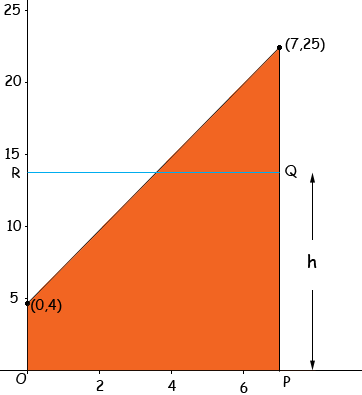Name: Justin Who is asking: Student Level of the question: All Question: Find the average value of the following function from x=0 to x=7 f(x)=3x+4 Hi Justin, I drew a graph of the function f(x) from x = 0 to x = 7 and shaded orange the region between the the graph of f(x), the x-axis and the lines x = 0 and x = 7. The average of the function over this interval is the height h so that the area of the region shaded orange is the same as the area of the rectangle OPQR.The area of the rectangle is 7h and the area of the rectangle you can find using geometry or calculus. Penny